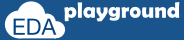## Contact / Report an issue

### functions argument passing

SystemC/C++ provides below means for passing arguments to functions,
• pass by value
• pass by reference

### pass by value

In argument pass by value, argument passing mechanism works by copying each argument into the subroutine area. if any changes to arguments with in the subroutine, those changes will not be visible outside the subroutine.

#### Example-1:

The example below shows argument pass by value.
variables x and y are passed as an argument in the function call sum, changes to the argument x with in the function is not visible outside.

```#include "systemc.h"

//function sum
int sum (int x,int y)
{
return x = x+y;
}

int sc_main (int argc, char* argv[]) {
int x=20;
int y=30;
int z;

//function call
z=sum(x,y);

//value of x,y and z
cout <<"Value of x="<<x<<endl;
cout <<"Value of y="<<y<<endl;
cout <<"Value of z="<<z<<endl;
// Terminate simulation
return 0;
}
```

#### Simulator Output:

Value of x=20
Value of y=30
Value of z=50
Execute the above code on### argument pass by reference

reference to the original argument is passed to the subroutine. as the argument with in subroutine is pointing to an original argument, any changes to the argument with in subroutine will be visible outside. To indicate argument pass by reference, the argument type is suffix with &.

#### Example-2

The example below shows argument pass by reference.
variables x and y are passed as an argument in the function call sum, changes to the argument x with in the function, is visible outside.

```#include "systemc.h"

//function sum
int sum (int& x,int& y)
{
return x = x+y;
}

int sc_main (int argc, char* argv[]) {
int x=20;
int y=30;
int z;

//function call
z=sum(x,y);

//value of x,y and z
cout <<"Value of x="<<x<<endl;
cout <<"Value of y="<<y<<endl;
cout <<"Value of z="<<z<<endl;
// Terminate simulation
return 0;
}
```

#### Simulator Output:

Value of x=50
Value of y=30
Value of z=50

Execute the above code on### const keyword

Any modifications to the argument value in pass by reference can be avoided by using const keyword before argument type, any attempt in changing the argument value in subroutine will lead to compilation error.

#### Example-3

The example below shows argument pass by reference with const keyword.
variables x and y are passed as an argument in the function call sum, as arguments are mentioned as const, changes to the argument x with in the function leads to compilation error.

```#include "systemc.h"

//function sum
int sum (const int& x,const int& y)
{
return x = x+y;
}

int sc_main (int argc, char* argv[]) {
int x=20;
int y=30;
int z;

//function call
z=sum(x,y);

//value of x,y and z
cout <<"Value of x="<<x<<endl;
cout <<"Value of y="<<y<<endl;
cout <<"Value of z="<<z<<endl;
// Terminate simulation
return 0;
}
```

#### Simulator Output:

testbench.cpp: In function 'int sum(const int&, const int&)':
testbench.cpp: error: assignment of read-only reference 'x'
Exit code expected: 0, received: 1
Execute the above code on### default argument values

default value can be specified to the arguments of subroutine.
In the subroutine call, arguments with default value can be omitted from the call. if any value is passed to an argument with default value, then the new value will be considered.

#### Example-4

The example below shows argument with default value.

```#include "systemc.h"

//function sum
int sum (int x=10,int y=20)
{
return x = x+y;
}

int sc_main (int argc, char* argv[]) {
int z;

//function takes defalut values for arguments
z=sum();
cout <<"Value of z="<<z<<endl;

//function takes default value for second argument
z=sum(20);
cout <<"Value of z="<<z<<endl;

// Terminate simulation
return 0;
}
```

#### Simulator Output:

Value of z=30
Value of z=40
Execute the above code on